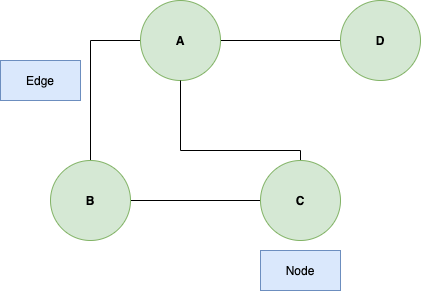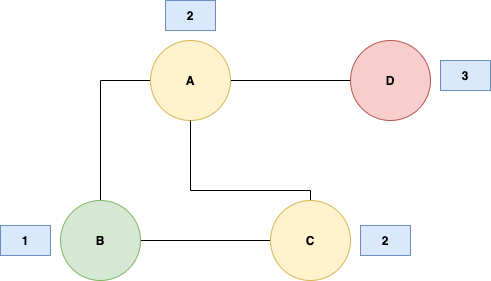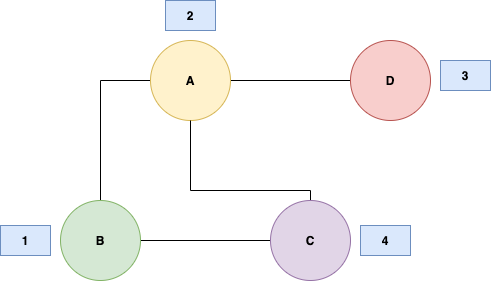# Audience

This article is aimed at developers with a small amount of experience implementing structures such as linked lists and trees, but who would like to continue their learning into graphs.

I will use Java, but the concepts should be approachable by engineers familiar with any language!

# Argument

We begin by introducing the concept of graphs. A graph is non-linear structure — unlike linked lists, queues, stacks and arrays. It consists of nodes and edges, as demonstrated below.An example graph with four nodes, connected by four edges.

By traversing the graph we can reach any of the nodes. We can also have graphs where not all nodes are connected. For those familiar with trees, we can see trees are a subset of graphs with extra restrictions.

There are two core types of graph: directed (also known as digraphs), and undirected. Digraphs have edges with a concept of direction, whereas undirected graphs do not. The above is an example of an undirected graph (where we can travel both ways round an edge), whereas the below is a digraph (where we can only move in one direction).

In the real world we may represent a friendship group as an undirected graph. Friendships are mutual, therefore if Jennifer is friends with Aiza, Aiza must also be friends with Jennifer. A digraph can be used to represent links on webpages. Google may link to Medium, but that doesn’t necessarily mean the reverse is true.

Another useful concept is the idea of weighted graphs, where edges are assigned a weight. We could graph cities and highways using this method, where the weight is the distance of the highway.

## Representing Graphs

Now we have covered graphs conceptually, we move onto how we might represent them as code. This is commonly done using an adjacency list. For each node we maintain a list of the nodes it connects to.

This represents the first graph we introduced. As we add connections from the source to the destination, and from the destination to the source, it is undirected. We can make it directed by removing a line and only adding connections from the source to the destination.

Now we have our graph represented in code let’s look at searching it.

## Breadth first search (BFS)

Breadth first search is where we visit each of the nodes connected to our current node, before moving on to the others. In our initial graph, we have the below ordering of visits.We start at node B, then visit nodes A and C, then node D

The above diagram represents a BFS starting at node B. We then visit nodes A and C, then finally node D. We can represent this in the coded solution below.

## Depth First Search

Depth first search is different in that we go all of the way to the end of a path before recursing back up and exploring the next one.Potential DFS routes in our graph

We start at `B`, then go through to `A`, then to `D`. This marks the end of our current possible routes, so we return to `B` and look for the next one. The only remaining node is `C`, so we follow that path all the way down. As we have seen `A` before, we do not visit it, and that’s us done!

A recursively coded solution is below.

We can also implement this in an iterative fashion, avoiding recursion.

## Common Problems

To understand graphs further, let’s look at a problem from LeetCode.

There are a total of `numCourses` courses you have to take, labeled from `0` to `numCourses - 1`. You are given an array `prerequisites` where `prerequisites[i] = [ai, bi]` indicates that you must take course `bi` first if you want to take course `ai`.

For example, the pair `[0, 1]`, indicates that to take course `0` you have to first take course `1`.

Return the ordering of courses you should take to finish all courses. If there are many valid answers, return any of them. If it is impossible to finish all courses, return an empty array.

To me, this suggests a digraph. If we need to take course `A` before course `B`, and course `B` before course `C`, then we can represent this using the below.